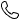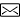0373-59399252851259250@qq.com# 柱筒中掺杂手性剂向列相液晶的介电及挠曲电性能

2 理论模型

Fig.1  （a） Concentric cylindrical cell model of nematic liquid crystals with chiral dopants； （b） Schematic diagram showing the cylindrical coordinate system.

n=cosαsinβer+sinαsinβeϕ+cosβez .

（1）

fdiel=−12ε0Δε(n⋅E)2 ,

（2）

Pflexo=e1n(∇⋅n)+e3(∇×n)×n ,

（3）

fflexo=−(E⋅P)=Urlnρ[e1(−1rcos2αsin2β+cosαsinαsin2βdαdr−12dβdrcos2αsin2β)+e3(1rsin2αsin2β+12sin2αsin2βdαdr−12cos2αsin2βdβdr)] .

（4）

felas=12[K11(∇⋅n)2+K22(n⋅∇×n+q0)2+K33(n×∇×n)2] ,

（5）

F=2π∫R2R1(felas+fdielec+fflexo)rdr.

（6）

K11{12sin2αsin2βd2βdx2−sin2αsin2β[1+(dαdx)2+(dβdx)2]−2sin2αsin2βdαdxdβdx−2sin2αsin2βd2αdx2}+K22{−12sin2αsin2βd2βdx2+2sin2αsin2β[(dβdx)2+sin2βdβdx]+sin2αsin2βcos2β⋅[1+(dαdx)2]−cos2αsin4βdαdxdβdx−2cos2αsin2βcos2βd2αdx2+4R1exq0cosαsin2βdβdx}+K33{sin2αsin4β[1+(dαdx)2]−sin2αsin2β[(dβdx)2+2sin2βdβdx]−2cos2αsin2β[2sin2βdαdxdβdx+d2αdx2sin2β]}+[ε0ΔεU2(lnρ)2+2U(e1+e3)lnρ]sin2αsin2β=0 .

（7）

K11{cos2αsin2β[1+(dβdx)2+(dαdx)2]−2cos2αcos2βd2βdx2+2sin2αcos2βdβdxdαdx+12sin2αsin2βd2αdx2}+K22{−cos2αsin2β(dαdx)2+sin2βcos2β[sin2α+cos2α(dαdx)2]−2sin2αd2βdx2−2sin2αdαdxdβdx−sin2αsin2β[2sin2βdαdx+12d2αdx2]−4R1q0exsinαsin2β−4R1q0excosαsin2βdαdx}+K33{2sin2βsin2β[sin2αdαdx+sin2α+cos2α(dαdx)2]+2sin2β[sin2αdβdxdαdx−cos2αd2βdx2]−cos2αsin2β(dβdx)2}−[ε0ΔεU2(lnρ)2+2Ue1lnρ]cos2αsin2β+2Ue3lnρsin2αsin2β=0 .

（8）

β(x=0)=0 , β(x=lnρ)=π ,

（9）

dαdx∣∣∣x=0=dαdx∣∣∣x=lnρ=0 .

（10）

3 数值计算与分析

3.1　介电效应的影响

Fig.2  Director distribution in cylinder cell without electric field

Fig.3  Director distribution when the flexoelectric effect is ignored. （a） nr（max） as a function of voltage； （b~d） Director components as functions of the normalized position under different voltages.

3.2　挠曲电效应的影响

Fig.4  Director components as functions of the normalized position for different values of flexoelectric coefficient e1， when U=0.9 V and e3=0， in which black， red and blue curves coincide. （a） nr； （b） nφ； （c） nz.

Fig.5  Director components as functions of the normalized position for different values of flexoelectric coefficient e3， when U=0.9 V and e1=0. （a） nr； （b） nφ； （c） nz.

3.3　挠曲电效应和介电效应的耦合作用

Fig.6  Director components as functions of the normalized position for different values of flexoelectric coefficient e1， when U=1.0 V and e3=0， in which black and red curves coincide. （a） nr； （b） nφ； （c） nz.

Fig.7  Director components as functions of the normalized position for different values of flexoelectric coefficient e3， when U=1.0 V and e1=0. （a） nr； （b） nφ； （c） nz

4 结 论

#### 栏目分类

【免责声明】：中州期刊联盟所提供的信息资源如有侵权、违规，请及时告知。